## Sankey Diagram - weight

Hi everybody,

i'm facing some problem understanding the usage of the weigth in sankey diagram on VA.

I have this kind of data

 Trans_ID Item Order Elapsed 1 A 1 120 1 B 2 120 1 C 3 180 1 D 4 30 2 A 1 120 2 B 2 30 3 A 1 60 3 A 2 60 4 B 1 120 4 A 2 60 4 C 3 30

I would like that the width of the path would represent the average of elapsed (used as weigth).

Is it possible? if yes, where i can change the aggregation measure for the weight.

Thank you

@FalkoSchulz  can you help me?

5 REPLIES 5

## Re: Sankey Diagram - weight

Hi!

Note, that aggregation is done on path level and not on segment (or event) level. Taking your example - it renders as follow in VA 8.x: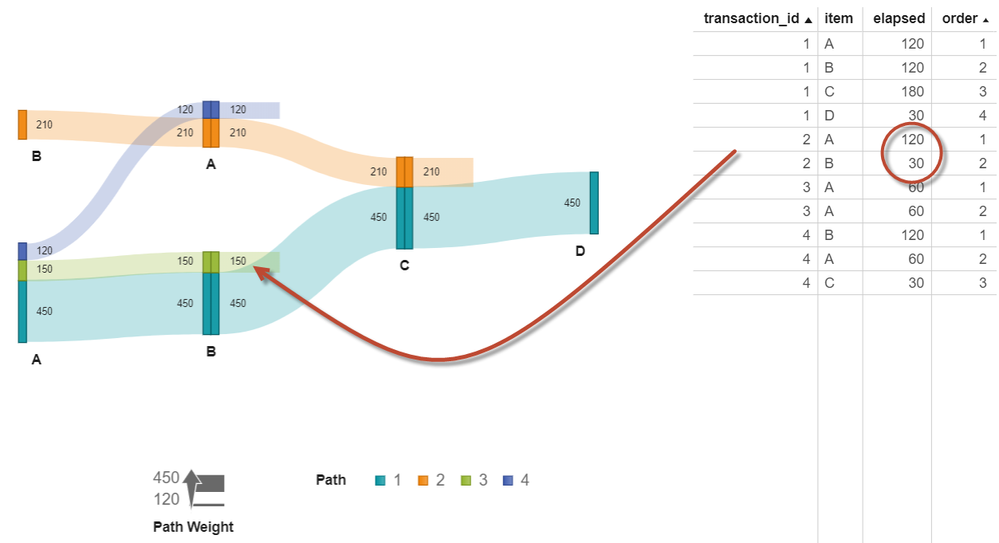Let's just look at one path for illustration purposes (A->B) as marked in above screenshot. Taking all events for this path the aggregated path weight is 150.

Let's add another transaction (#5) for the same path and it renders as: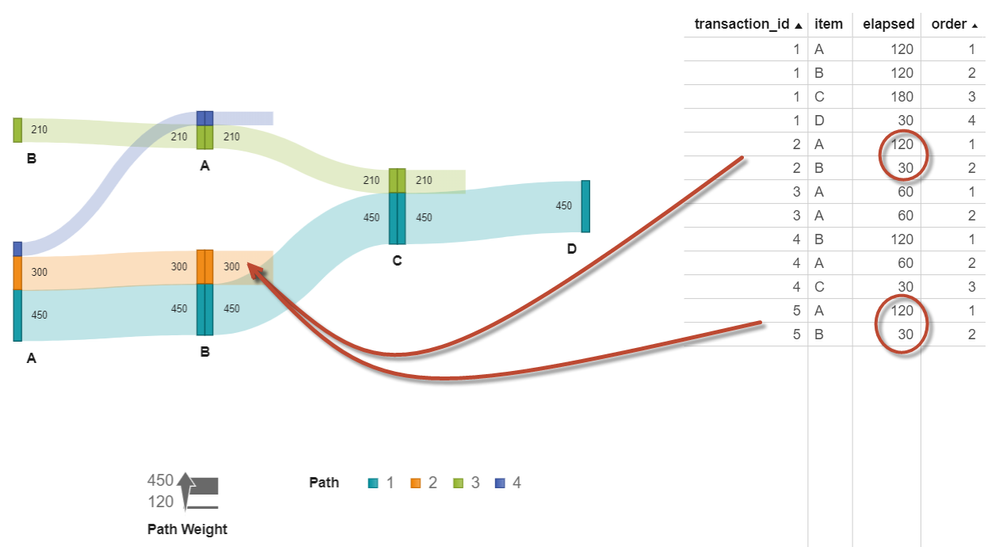The total weight of the same path is now 300 given the two contributing transactions (#2 and #5). If you want to see the average across all paths instead (so 150 in the example above) - you would change the aggregation of the weight variable in the data panel: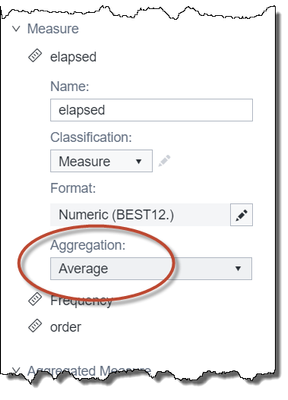Hope this helps. Regards, Falko

## Re: Sankey Diagram - weight

if i understand correctly i have to change the aggregation method in the data properties in the lower left of the screen.

So it is not referring to diagram properties.

If it is, i can also set Median for the aggregation methods.

Is it correct?

Thank you

## Re: Sankey Diagram - weight

Hi,

Yes, that is correct - the applied aggregation is on data item level so something you adjust in the data panel. As per documentation - supported aggregations for the weight role include sum, average, minimum, or maximum.

Hope this helps. Regards, Falko

## Re: Sankey Diagram - weight

Hi @FalkoSchulz ,

I would ask you something about the diagram representation differences based on the weight aggregation you use.

the data are the same as i reported above.

If i use frequency as measure to determine the width of the path i have several path above 2 event (since i specified minimum path length = 2).

let's say i choose SUM as weight aggregation and i still have several path with more than 2 events.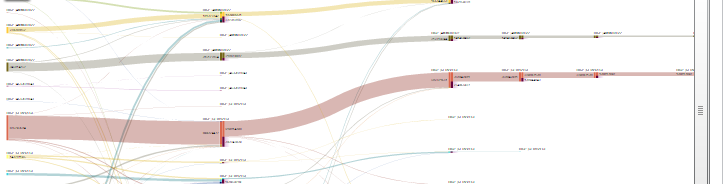If i choose AVARAGE the diagram shows me only paths with just 2 events.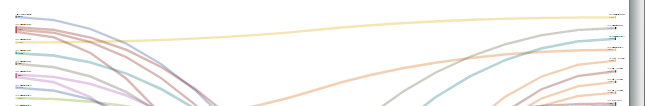I'm wondering why it could happen.

Thank you

## Re: Sankey Diagram - weight

Sorry for the delay in responding. As far as I remember the graph shouldn't change (in terms of number of paths displayed) if you change the aggregation. Are you able to share your data so I can see more details (the screens you shared indicate you have used a different data set than the one used in the first example). Or alternatively - can you try using the original data and see whether you see similar results?

Thanks, Falko

Discussion stats
• 5 replies
• 1050 views
• 0 likes
• 2 in conversation